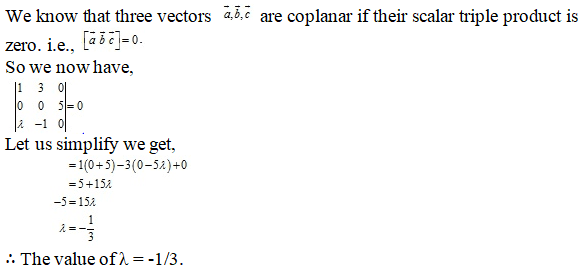# RD Sharma Solutions Class 12 Scalar Triple Product Exercise 26.1

RD Sharma Solutions for Class 12 Maths Chapter 26 Scalar Triple Product Exercise 26.1, is provided here for students to prepare for exams. These solutions are prepared by experts to help students prepare for their board exams at ease. Practising RD Sharma Class 12 Solutions help students to score good marks in the exam. Pdf consisting of the exercise solutions can be easily downloaded from the links provided below.

## Download PDF of Rd Sharma Solution for Class 12 Maths Chapter 26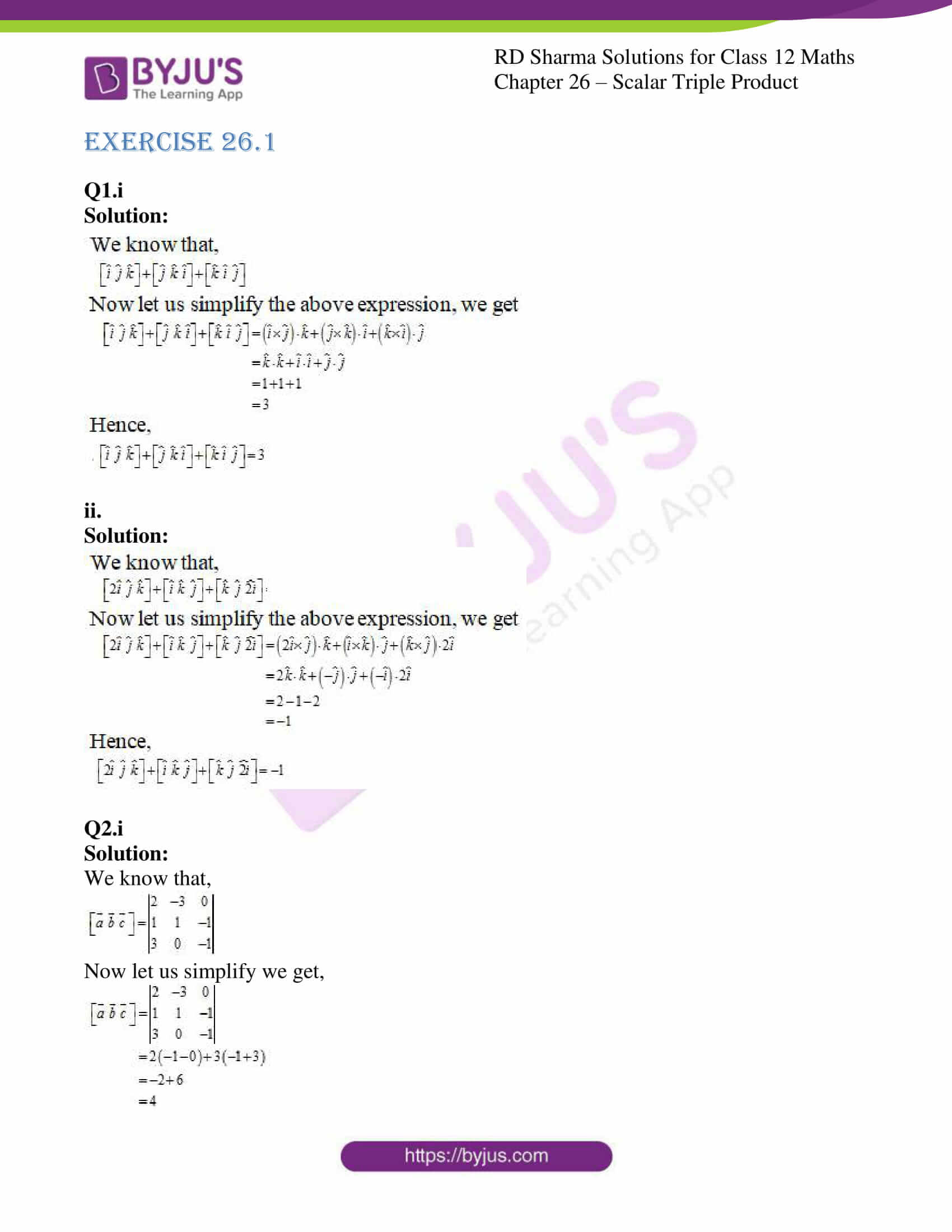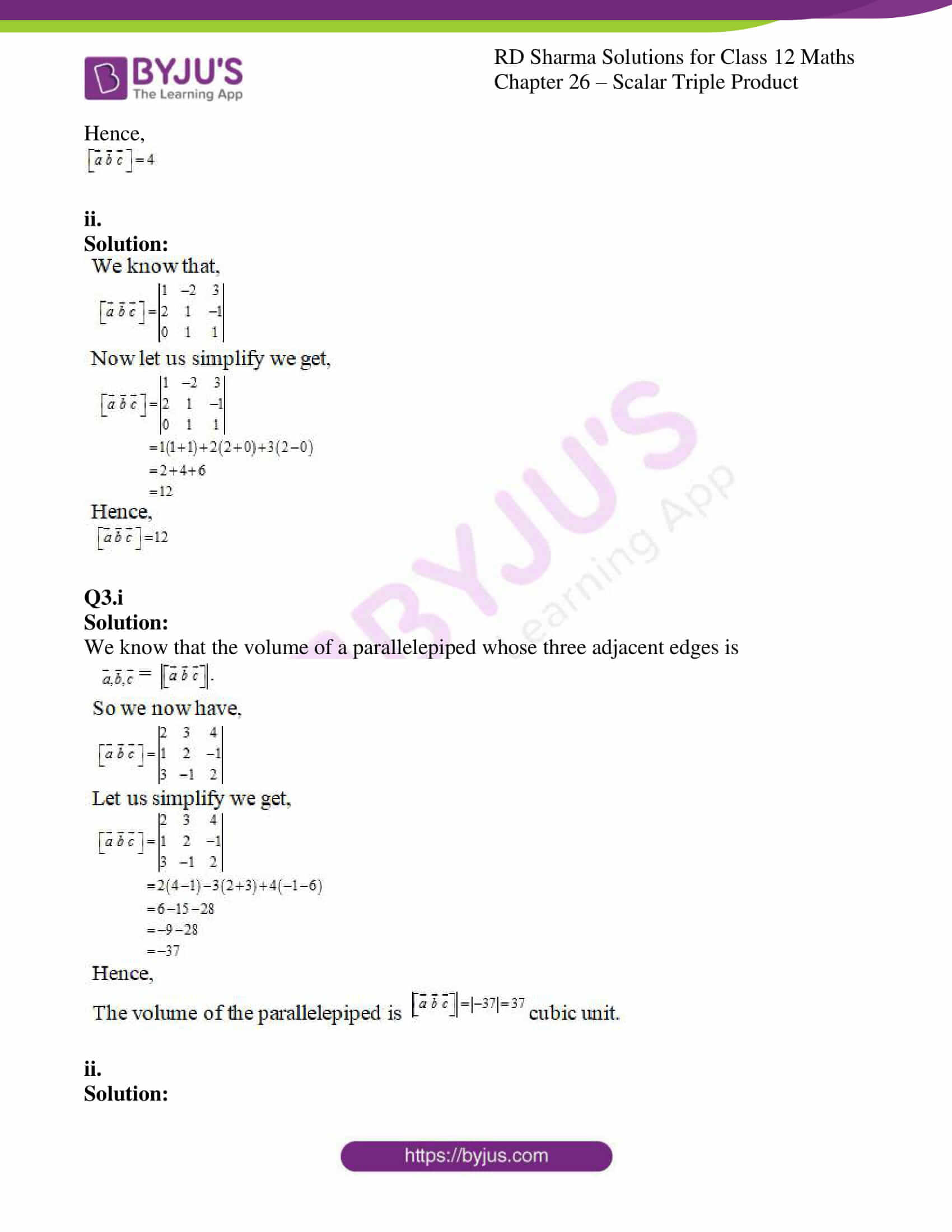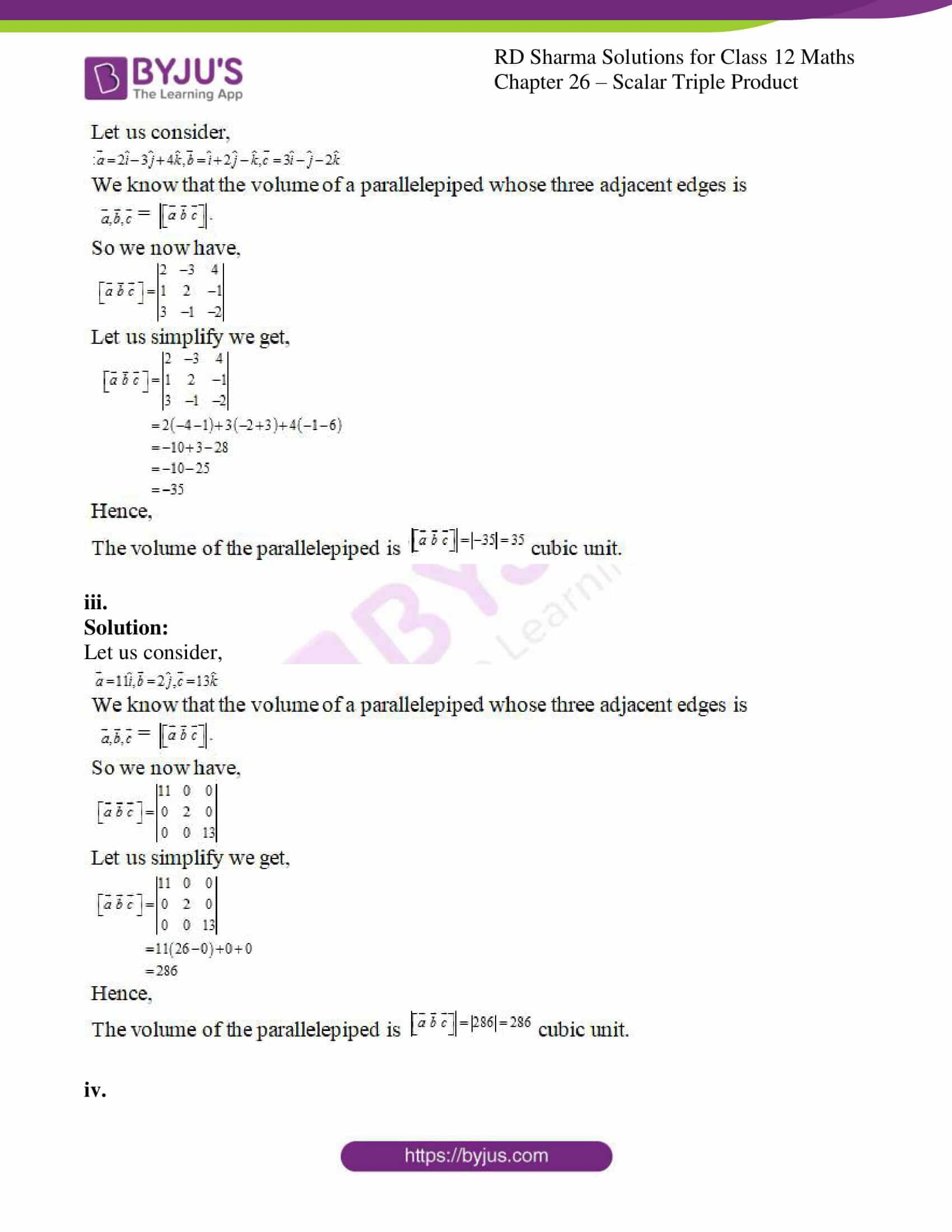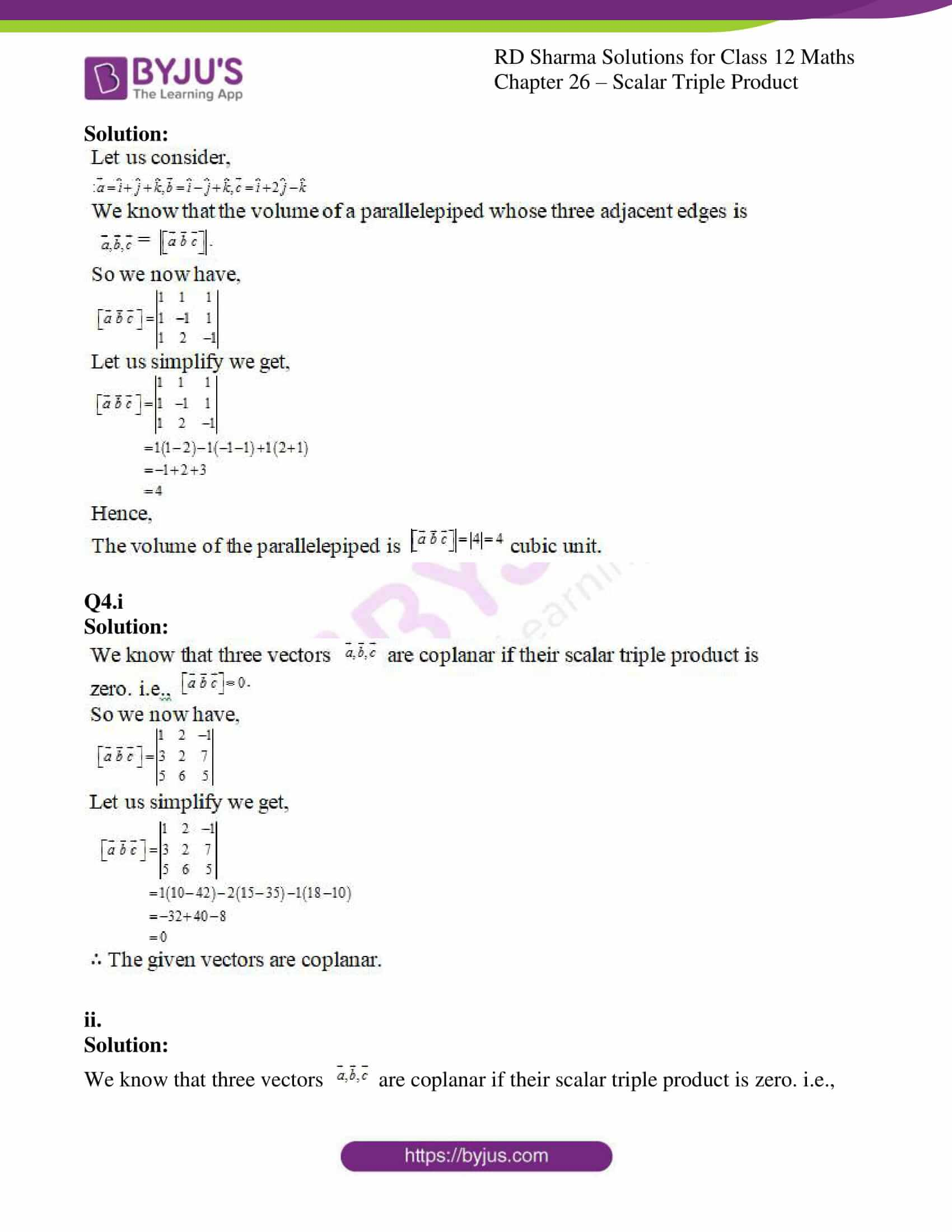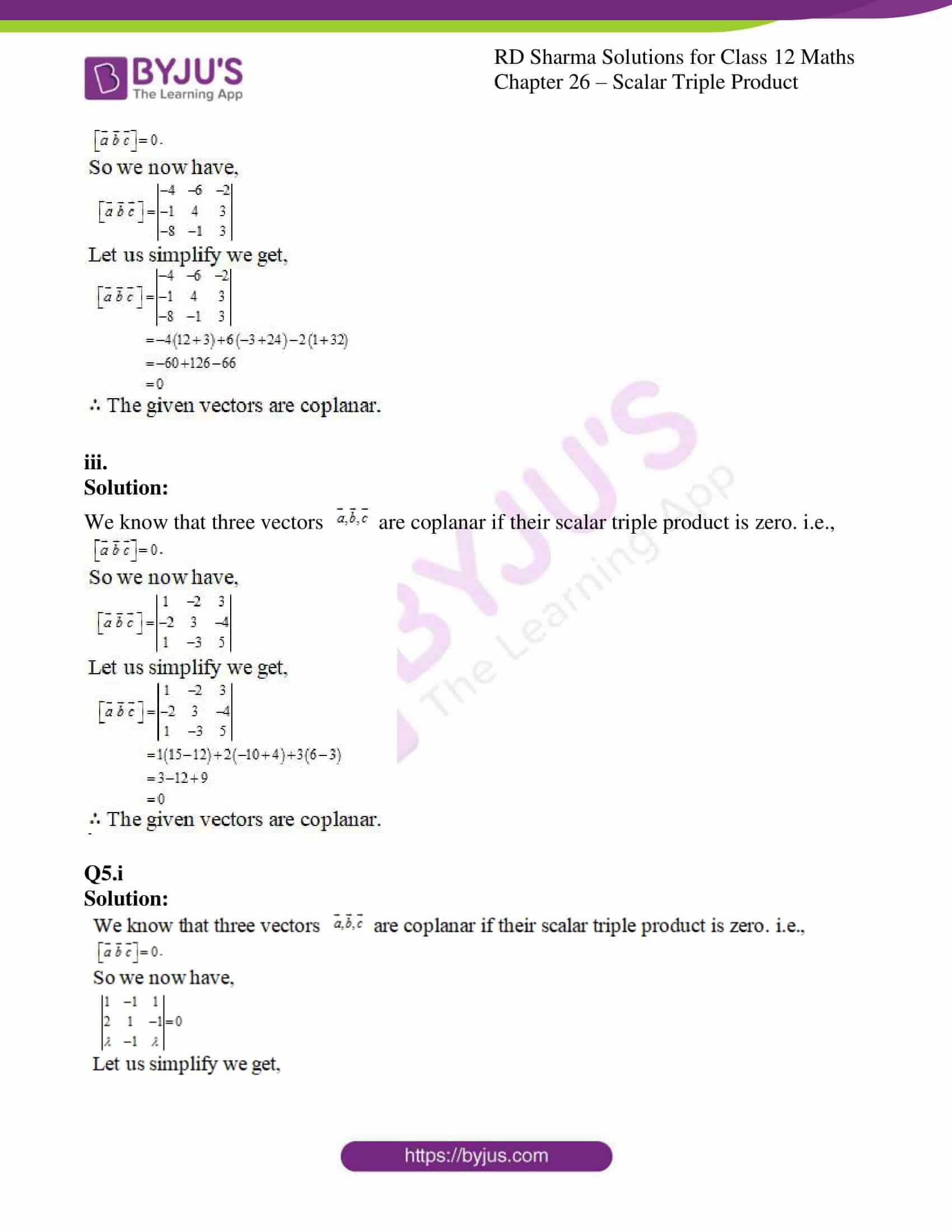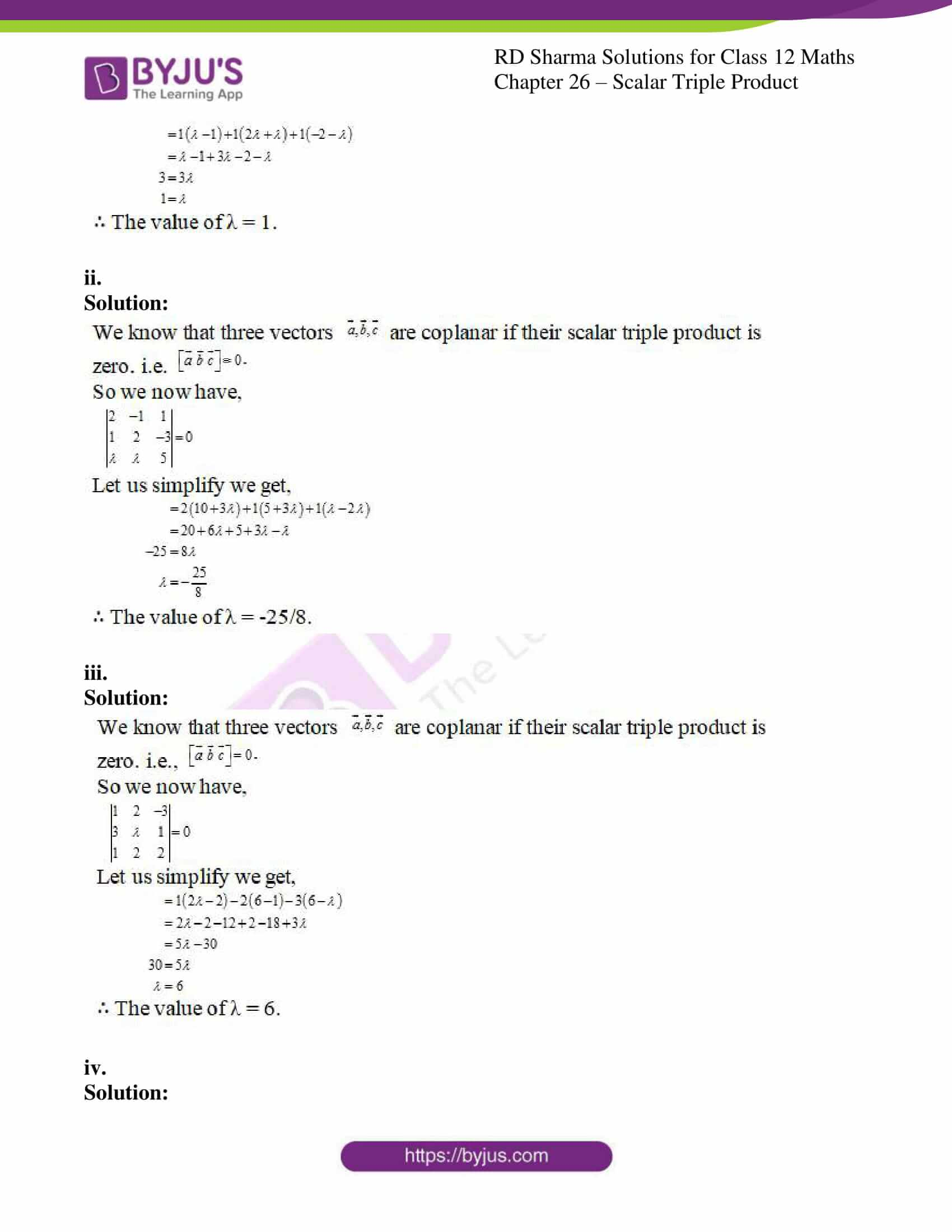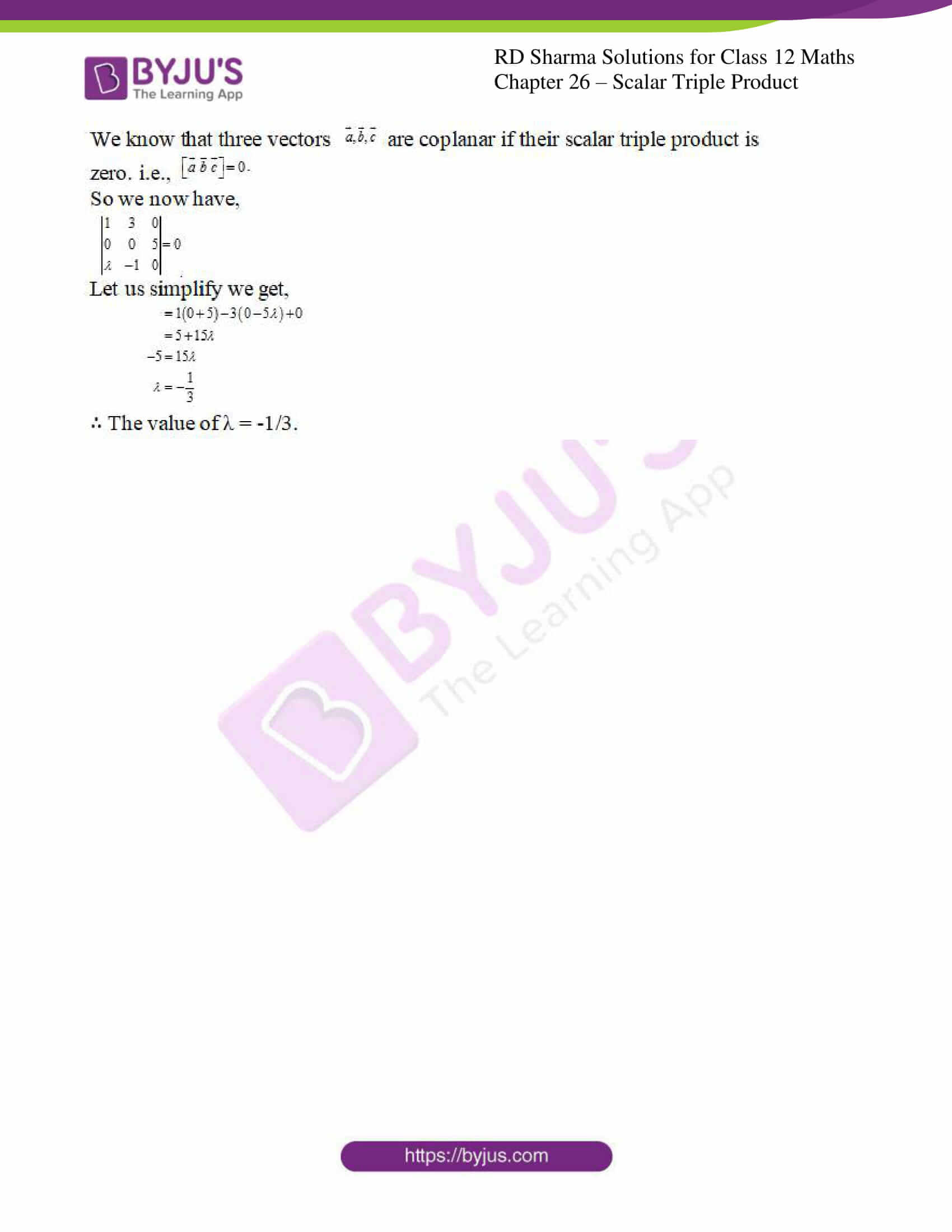### Access Answers for Rd Sharma Solution Class 12 Maths Chapter 26

EXERCISE 26.1

Q1.i

Solution: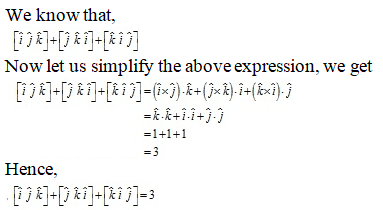ii.

Solution: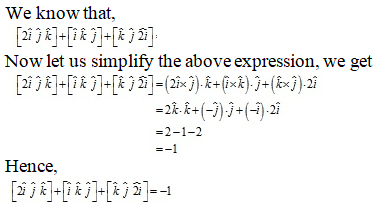Q2.i

Solution:

We know that,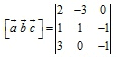Now let us simplify we get,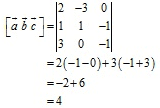Hence,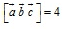ii.

Solution: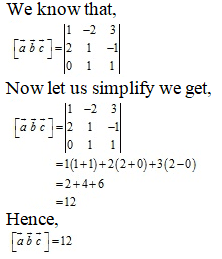Q3.i

Solution:

We know that the volume of a parallelepiped whose three adjacent edges is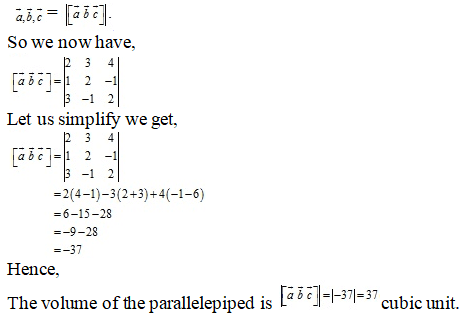ii.

Solution: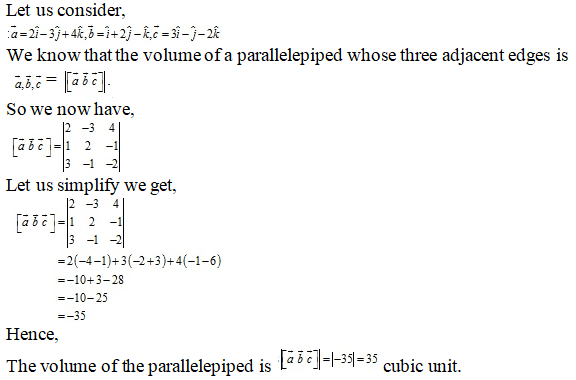iii.

Solution:

Let us consider,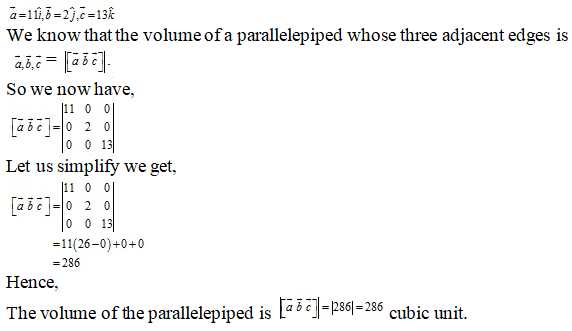iv.

Solution: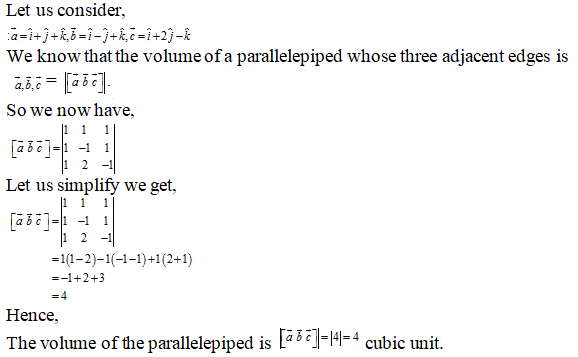Q4.i

Solution: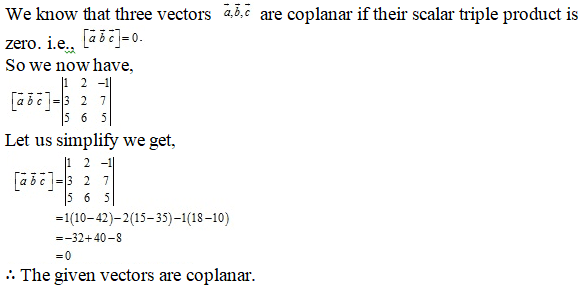ii.

Solution:

We know that three vectors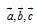are coplanar if their scalar triple product is zero. i.e.,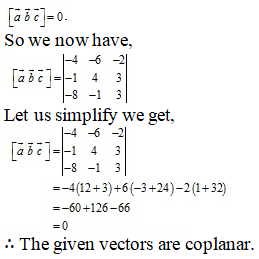iii.

Solution:

We know that three vectorsare coplanar if their scalar triple product is zero. i.e.,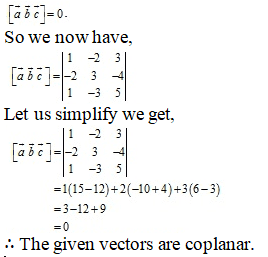Q5.i

Solution: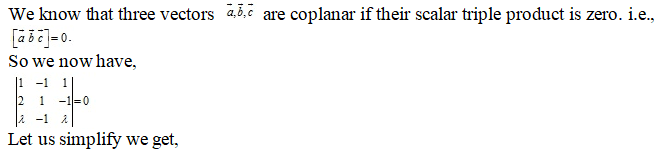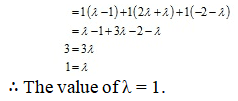ii.

Solution: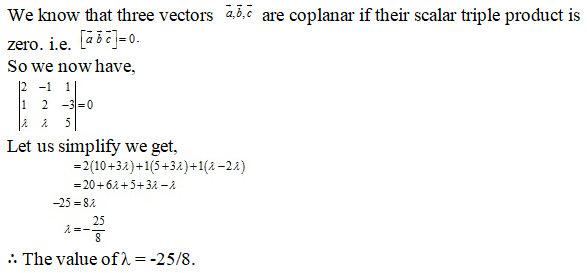iii.

Solution: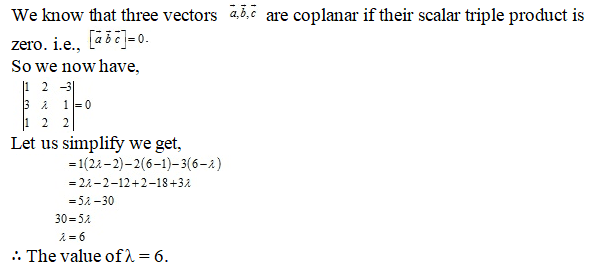iv.

Solution: